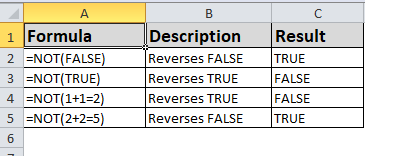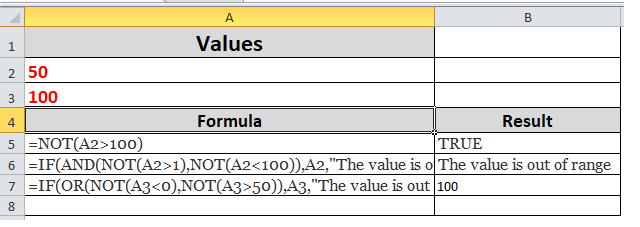## Excel Office

Excel How Tos, Tutorials, Tips & Tricks, Shortcuts

# NOT function: Description, Usage, Syntax, Examples and Explanation

## What is NOT function in Excel?

NOT function is one of the Logical functions in Microsoft Excel that is used when you want to make sure one value is not equal to another.

## Examples of NOT function## Examples

Here are some general examples of using NOT by itself, and in conjunction with IF, AND and OR.

Also See:   Highlight column differences in ExcelFormula Description =NOT(A2>100) A2 is NOT greater than 100 =IF(AND(NOT(A2>1),NOT(A2<100)),A2,”The value is out of range”) 50 is greater than 1 (TRUE), AND 50 is less than 100 (TRUE), so NOT reverses both arguments to FALSE. ANDrequires both arguments to be TRUE, so it returns the result if FALSE. =IF(OR(NOT(A3<0),NOT(A3>50)),A3,”The value is out of range”) 100 is not less than 0 (FALSE), and 100 is greater than 50 (TRUE), so NOT reverses the arguments to TRUE/FALSE. ORonly requires one argument to be TRUE, so it returns the result if TRUE.
Also See:   How to use Excel NOT Function